# Algorithm -- Permutation Combination Subset

Given a collection of numbers, return all possible Permutations, K-Combinations, or all Subsets are the most fundamental questions in algorithm.

They can be impelmented by simple recursion, iteration, bit-operation, and some other approaches. I mostly use Java to code in this post.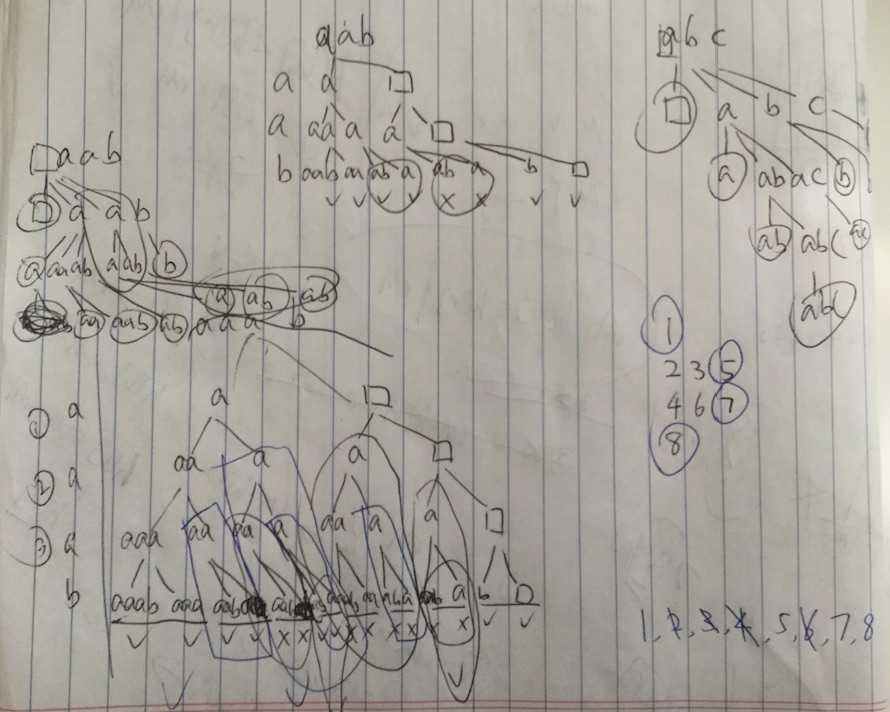## Permutation

Given a collection of numbers, return all possible permutations.
For example,
[1,2,3] have the following permutations:
[1,2,3], [1,3,2], [2,1,3], [2,3,1], [3,1,2], and [3,2,1].

also see: CrackingCoding: C9Q5, LeetCode: Permutations

Time Complexity: $$O(n!)$$

### Recursion – DFS

The idea of iteration to solve this problem is dervied from Depth First Search (DFS).Along with the increasing of recursing depth, the amount number of subnodes of each node is decreasing by one. This is why the time complexity is $$O(n!)$$.

public List<String> perm(int[] nums) {
List<int[]> result = new ArrayList();
if (nums = null) return null;
perm(nums, 0, result);
return result;
}

public void perm(int[] nums, int start, List<int[]> result) {
if (start == nums.length) {
return;
}

for (int i = start; i < nums.length; i++) {
swap(nums, start, i);
perm(nums, start + 1, result);
swap(nums, start, i);
}
}

public void swap(int[] nums, int start, int end) {
int tmp = nums[start];
nums[start] = nums[end];
nums[end] = tmp;
}


### Recursion – DFS, Permutation II

What if there are some duplicated characters in the given set? We can modify the previous algorithm to achieve the new solution.

Use a HashSet<Character> to remember whether a Char has been swap or not.

Set<Character> has = new HashSet();
for (int i = st; i < set.length; i++) {
swap(set, st, i);
permutations(set, st + 1, res);
swap(set, st, i);
}
}


Where has.add(set[i]) will return FALSE is set[i] is already in the has.

### Recursion – Generated by Permutation(n-1)

The same solution as that of CrackingCoding. Insert the current number at every possible position into each of the last permutations.

public List<List<Integer>> permute(int[] num) {
if (num == null || num.length == 0) {
return new ArrayList<List<Integer>>();
}
return permute(num, num.length - 1);
}

public List<List<Integer>> permute(int[] num, int ed) {
List<List<Integer>> res = new ArrayList<List<Integer>>();
if (ed == 0) {
List<Integer> one = new ArrayList<Integer>();
return res;
}

List<List<Integer>> lastRes = permute(num, ed - 1);
for (List<Integer> lastOne : lastRes) {
for (int i = 0; i < lastOne.size(); i++) {
List<Integer> one = new ArrayList<Integer>(lastOne);
lastOne.remove(i);
}
}
return res;
}


### Iteration – Next Permutation

The iteration idea is derived from a solution for Next Permutation. It could also be used to solve Unique Permutation, while there are duplicated characters existed in the given array.

For example,
[1,1,2] have the following unique permutations:
[1,1,2], [1,2,1], and [2,1,1].

The function of nextPermutation(int[] num) is used to generate the smallest permutation among the possible permutations which are greater than the given int[] num in numeric concept.

Here are some examples of nextPermutation
1,2,3 → 1,3,2
3,2,1 → 1,2,3
1,1,5 → 1,5,1
public List<List<Integer>> permuteUnique(int[] num) {
Arrays.sort(num);
List<List<Integer>> ans = new ArrayList<List<Integer>>();
do {
List<Integer> l = new ArrayList<Integer>();
for (int n : num) {
}
} while (nextPermutation(num));
return ans;
}

private boolean nextPermutation(int[] num) {
if (num.length <= 1) {
return false;
}
int i = num.length - 1;
while (true) {
i--;
if (num[i] < num[i + 1]) {
int j = num.length;

while (num[i] >= num[--j]) {}
// !!! This non-content loop is useful to set the position of j,
// UNTIL num[i] < num[j]

swap(num, i, j);
reverse(num, i + 1, num.length);
return true;
}
if (i == 0) {
reverse(num, 0, num.length); //!!! back to the first smallest array
return false;
}
}
}

private void swap(int[] num, int i, int j) {
int temp = num[i];
num[i] = num[j];
num[j] = temp;
}

private void reverse(int[] num, int begin, int end) {
end--;
while (begin < end) {
swap(num, begin++, end--);
}
}


## Combination

Given two integers n and k, return all possible combinations of k numbers out of 1 ... n.
For example,
If n = 4 and k = 2, a solution is:
[ [2,4], [3,4], [2,3], [1,2], [1,3], [1,4] ]

also see: LeetCode: Combinations

Time Complexity: $$O(2^n)$$ without triming branches, $$O(2^k)$$ with triming.

### Recursion – DFSpublic List<List<Integer>> combine(int n, int k) {
List<List<Integer>> result = new ArrayList<List<Integer>>();
combine(0, n, k, new ArrayList<Integer>(), result);
return result;
}

public void combine(int depth, int n, int k,
List<Integer> r, List<List<Integer>> result)
{
if(r.size()==k)
{
return;
}
if(depth == n) return;

combine(depth + 1, n, k, r, result);
r.remove(r.size() - 1);
combine(depth + 1, n, k, r, result);
}


### Recursion – Generated by Last Combination

e.g. combine(4,2):
depth == 0: [ ]
depth == 1: , , , 
depth == 2: [1,2], [1,3], [1,4], [2,3], [2,4], [3,4]

/* n is total numbers length: 1..n
depth is current recursion depth
k is final, used to trim branches */
public List<List<Integer>> combine(int n, int depth, final int k) {
List<List<Integer>> result = new ArrayList<List<Integer>>();

if (depth == 0) {
List<Integer> empty = new ArrayList<Integer>();
return result;
}

List<List<Integer>> preRes = combine(n, depth - 1, k);
for (List<Integer> one : preRes) {
int last = one.isEmpty() ? 0 : one.get(depth - 2);
for (int i = last + 1; i <= n; i++) {
if (n - i + depth < k) { break; } // trim branches
List<Integer> newOne = new ArrayList<Integer>(one);
}
}
return result;
}


## Subset

Given a set of distinct integers, S, return all possible subsets.

also see: CrackingCoding: C9Q4, LeetCode: Subsets

Time Complexity: $$O(2^n)$$

### Recursion – DFS

DFS of Subset is similar to that of Combination. Actually, Subset problem is to get all Combination from [n,0] to [n,n].

That is, NO triming branches during recursion. Retrieving all the results when recurion depth == S.length.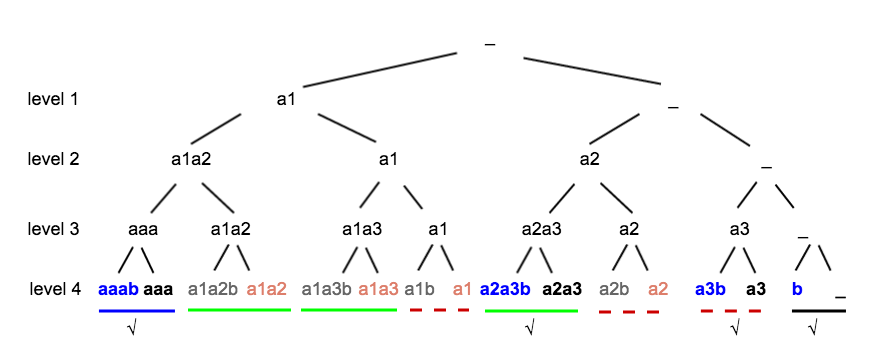public List<List<Integer>> subsets(int[] S) {
int len = S.length;
List<List<Integer>> result = new ArrayList<List<Integer>>();
subsets(S, 0, new ArrayList<Integer>(), result);
return result;
}

private void subsets(int[] S, int depth, List<Integer> r, List<List<Integer>> result) {
if (S.length == depth) {
return;
}

subsets(S, depth + 1, r, result);
r.remove(r.size() - 1);             //!!! MUST have; otherwise
subsets(S, depth + 1, r, result);
}


Or, there is another recursion approach of recursion with inner loop: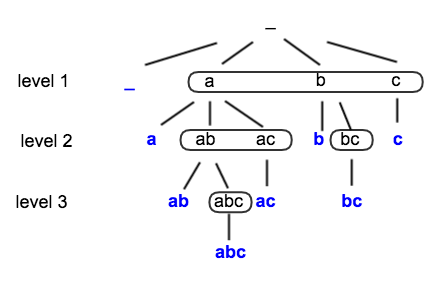private void subSets(int[] set, int depth, Deque<Integer> curr, List<List<Integer>> result) {
for (int i = depth; i < set.length; i++) {
curr.offerLast(set[depth]);
subSets(set, depth + 1, curr, result);
curr.pollLast();
}
}

• MUST have: becuase once [] hit the return and the recursion back to add level 2 (which adding 3 into []), the 3 will be never removed from [] object.

### Recursion – Generated by Subsets(n -1)

Generating Subsets(n): compute Subsets(n-1), clone the results, and then add $$a_n$$ to each of these cloned sets.

e.g. Subset(3)
Base case n = 0: []
Case n = 1: [], [a1]
Case n = 2: [], [a1], [a2], [a1,a2]
Case n = 3: [], [a1], [a2], [a1,a2], [a3], [a1,a3], [a2,a3], [a1,a2,a3]

public List<List<Integer>> getSubsets(List<Integer> set, int index) {
List<List<Integer>> allsubsets;
if (set.size() == index) { // Base case - add empty set
allsubsets = new ArrayList<List<Integer>>();
} else {
allsubsets = getSubsets(set, index + 1);
int item = set.get(index);
List<List<Integer>> moresubsets = new ArrayList<List<Integer>>();
for (List<Integer> subset : allsubsets) {
List<Integer> newsubset = new ArrayList<Integer>();
}
}
return allsubsets;
}


And there is another approach:

public List<String> subSets(String set) {
List<String> res =  new ArrayList<>();
if (set == null) return res;
subSets(set, 0, new StringBuilder(), res);
return res;
}

private void subSets(String set, int st, StringBuilder sb, List<String> res) {
if (st == set.length()) {
return;
}
subSets(set,st + 1, sb, res);
sb.append(set.charAt(st));
subSets(set, st + 1, sb, res);
sb.deleteCharAt(sb.length() - 1);
}


### Iteration – Similar Logic as Previous, more Space Efficient

public List<List<Integer>> subsets(int[] num) {
Arrays.sort(num);
List<List<Integer>> subsets = new ArrayList<List<Integer>>();
for (int i = 0; i < num.length; i++) {
int prevLast = subsets.size(); //!!!
for (int j = 0; j < prevLast; j++) {
List<Integer> subset = new ArrayList<Integer>(subsets.get(j));
}
}
return subsets;
}


### Binary Operation

All subsets problem could be described as a unique problem: generating each one set from a number among 0 to $$2^n$$, where n is the number of given set. Each set and number are one to one mapping.

explain: in order to get subsets from {1,2,3}, we need to do following choices when generating each one set:
pick {1} or not pick {1}
pick {2} or not pick {2}
pick {3} or not pick {3}
So, there are $$2^3$$ possibilities altogether, exactly, the amount of subsets.

Each of those choices could be considered as a binary operation choice: pick is 1, not pick is 0.
Then, {} could be represented as $$000_2 == 0_{10}$$, {1} as $$100_2 = 4_{10}$$, {1,3} as $$101_2 == 5_{10}$$, {1,2,3} as $$111_2 == 7_{10}$$.

public List<List<Integer>> subsets(int[] num) {
List<List<Integer>> set = new ArrayList<List<Integer>>();
for (int i = 0; i < 1 << num.length; i++) {
List<Integer> one = generateOne(i, num);
}
return set;
}

public List<Integer> generateOne(int k, int[] num) {
List<Integer> set = new ArrayList<Integer>();
for (int i = 0; i < num.length; i++) {
if ((k & (1 << i)) != 0) {
}
}
return set;
}


### Subsets in Range [a,b]

Actually, this problem could also be described as retrieving Combinations (n,a), (n,a+1) … (n,b).

We can generate those Combinations one by one, using same apporaches in Combination; or here is another choise: binary operation.

Remember in the last approach of Subset, we generate all the subsets using numbers from 0 ~ $$2^n$$. During these numbers, should we have a function to judge how many 1's is in each number, we could generating Subsets in ranger [a,b] by checking number of 1's is in ranger [a,b].

public List<List<Integer>> subsetsInRange(int[] num, int a, int b) {
List<List<Integer>> set = new ArrayList<List<Integer>>();
for (int i = 0; i < 1 << num.length; i++) {
int numberOf1bit = numberOf1(i);
if (numberOf1bit >= a && numberOf1bit <=b) {
List<Integer> one = generateOne(i, num);
}
}
return set;
}

/*ref: http://codercareer.blogspot.com/2011/11/no-20-number-of-1-in-binary.html */
public int numberOf1(int n)
{
int count = 0;
while (n != 0)
{
++ count;
n = (n - 1) & n;
}
return count;
}


### With Duplicated Characters

#### Subsets with Duplicated Characters

also see: LeetCode: Subsets II

According to Subsets recursion tree I:

There are more than one options to generate the unique subsets.

Here is one of them:

We keep left children (which means append the current level element); and discard those right children (not append) on condition that the current level element is same as the last element in the parent recursion result.

• According to Subset recursion tree I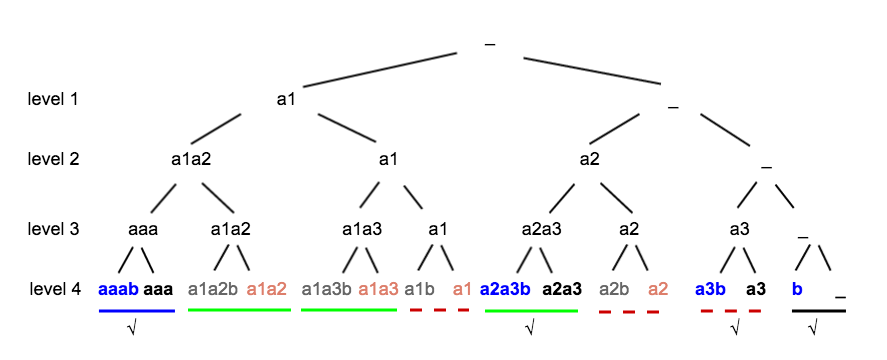private void subSets(char[] set, int st, Deque<Character> curr, List<String> res) {
if (st == set.length) {
return;
}
curr.offerLast(set[st]);
subSets(set, st + 1, curr, res);
curr.pollLast();
if (curr.isEmpty() || set[st] != curr.peekLast()) {
subSets(set, st + 1, curr, res);
}
}


Or,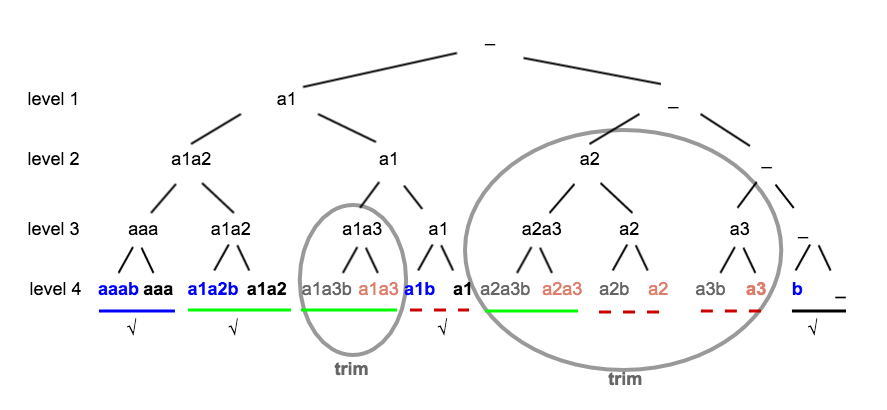private void subSets(char[] set, int st, Deque<Character> curr, List<String> res) {
if (st == set.length) {
return;
}
curr.offerLast(set[st]);
subSets(set, st + 1, curr, res);
curr.pollLast();
// Skips all the rest of duplicated letters (e.g. a1, a2, ... in this example)
while (st < set.length - 1 && set[st + 1] == set[st]) {
st++;
}
subSets(set, st + 1, curr, res);
}

• According to Subsets recursion tree II:

There are two options to generate the unqiue subsute:

1. Use a Set to avoid adding same element in each loop;

2. Judge if the current element is as same as the previous one inside each loop.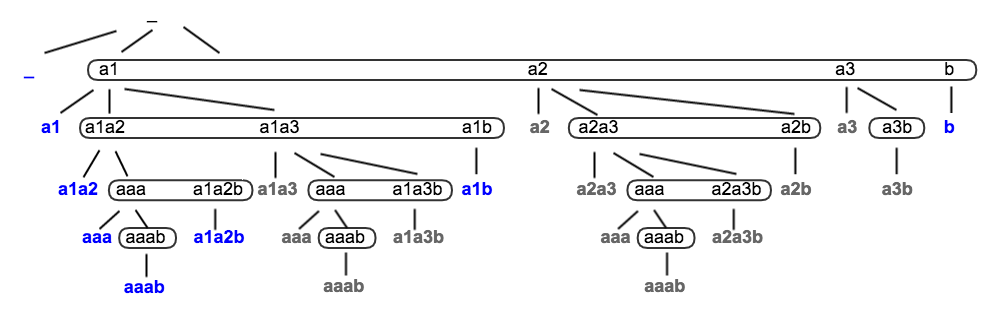//OR, Set<Character> mark = new HashSet();
for (int i = st; i < set.length; i++) {
if (i > st && set[i] == set[i-1]) { //OR, mark.contains(set[i])
continue;
}
curr.offerLast(set[i]);
subSets(set, i + 1, curr, res);